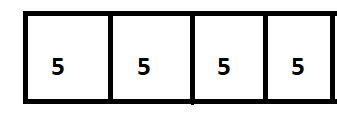# Math in Focus Grade 2 Chapter 16 Answer Key Using Bar Models: Multiplication and Division

This handy Math in Focus Grade 2 Workbook Answer Key Chapter 16 Using Bar Models: Multiplication and Division detailed solutions for the textbook questions.

## Math in Focus Grade 2 Chapter 16 Answer Key Using Bar Models: Multiplication and Division

Math Journal

Fill in the blanks, circles, and ovals.

To show 3 groups of 6 strawberries,
Step 1: I draw __________ strips of equal lengths in a row to represent 3 groups.
Step 2: I write the number ________ in the oval above one strip and a _________ in the oval below the strips.
Step 3: I write the number sentence.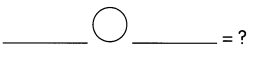Step 4: I _________ to find the answer.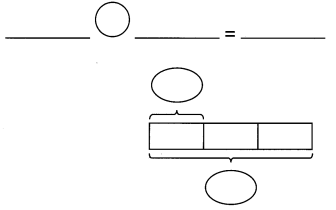Challenging Practice

Macy has some ropes, each 2 feet long. Nellie has some ropes, each 3 feet long. Oscar has some ropes, each 5 feet long. What is the length of each line of ropes when they are of the same length?

Problem Solving

Sheena bakes 100 muffins. She shares them equally with 4- other girls. How many muffins does each girl get? How many more muffins are needed so that each one gets two more muffins?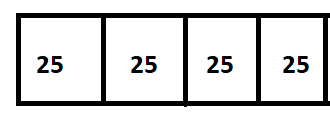So, 100 ÷ 4 = 25
Therefore, each girl gets 26 muffins.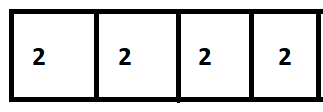So, 4 x 2 = 8
Therefore, 8 muffins are needed so that each one gets two more muffins.

Chapter Review/Test

Concepts and Skills

Match each bar model to a word problem. Then solve the problem.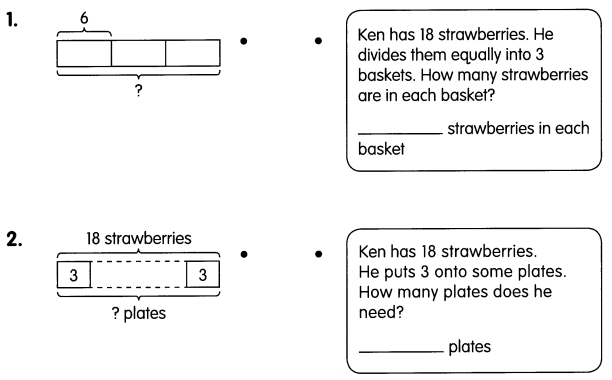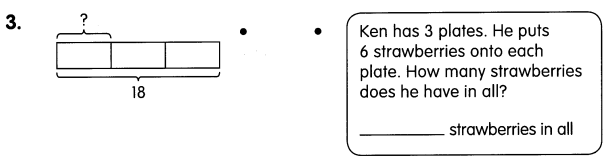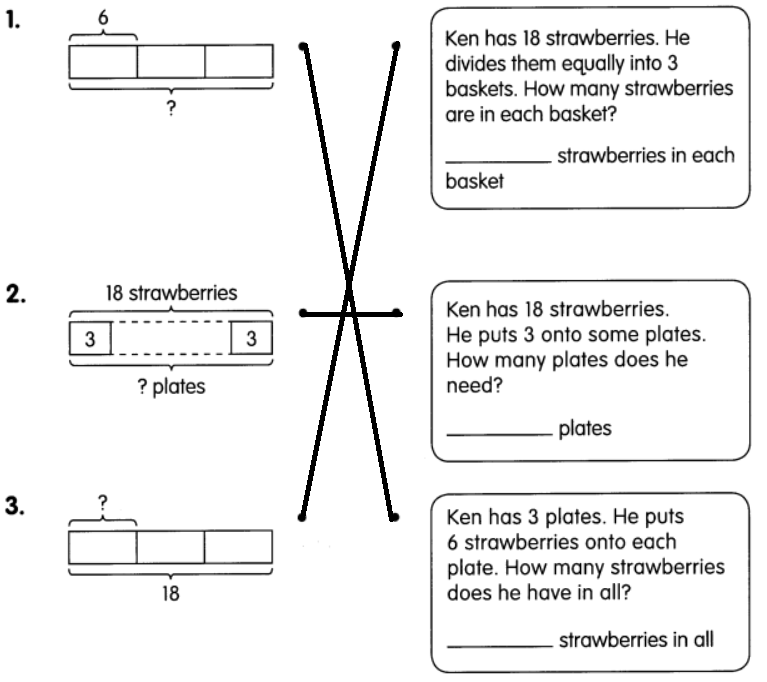Problem Solving

Write whether you need to multiply or divide. Then solve. Draw bar models to help you.

Question 4.
Jason has 5 apricots on each tray. He has a total of 50 apricots. How many trays are there?
There are ___________ trays.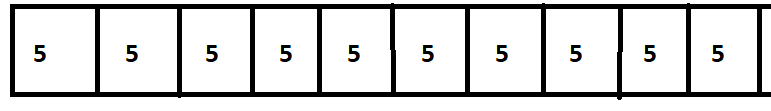So, 50 ÷ 5 = 10
Therefore, there are 10 trays.

Question 5.
There are __________ baskets in all.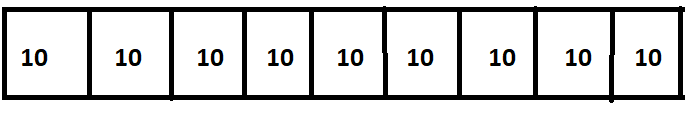So, 90 ÷ 10 = 9
Therefore, there are 9 baskets in all.

Write whether you need to multiply or divide. Then solve. Draw bar models to help you.

Question 6.
Sonny helps his mother pack old books in 6 boxes. Each box has a mass of 4- kilograms. What is the total mass of 6 boxes of books?
The total mass of 6 boxes of books is ___________ kilograms.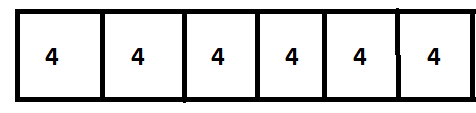So, 6 x 4 = 24
Therefore, the total mass of 6-boxes is 24 kilograms.

Question 7.
Sarah is making necklaces for her friends. She needs 12 feet of string for the necklaces. Each necklace needs a string 2 feet long. How many necklaces is Sarah making?
Sarah is making ___________ necklaces.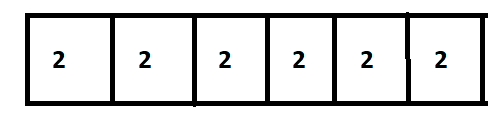So, 12 ÷ 2 = 6
Therefore, Sarah is making 6 necklaces.

Tell whether you need to multiply or divide. Then solve. Draw bar models to help you.

Question 8.
Billy’s cats drink 4- liters of milk in a week. How much milk do the cats drink in 4 weeks?
The cats drink ___________ liters of milk.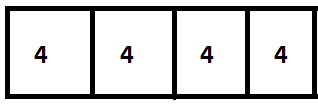Mr. Andres has 4 grandsons. He gives each of them $5. How much does he give his grandsons in all? He gives$___________ to his grandsons.